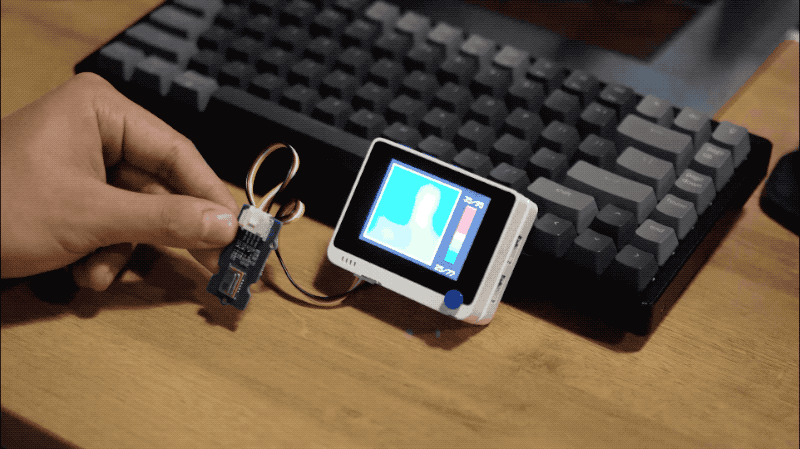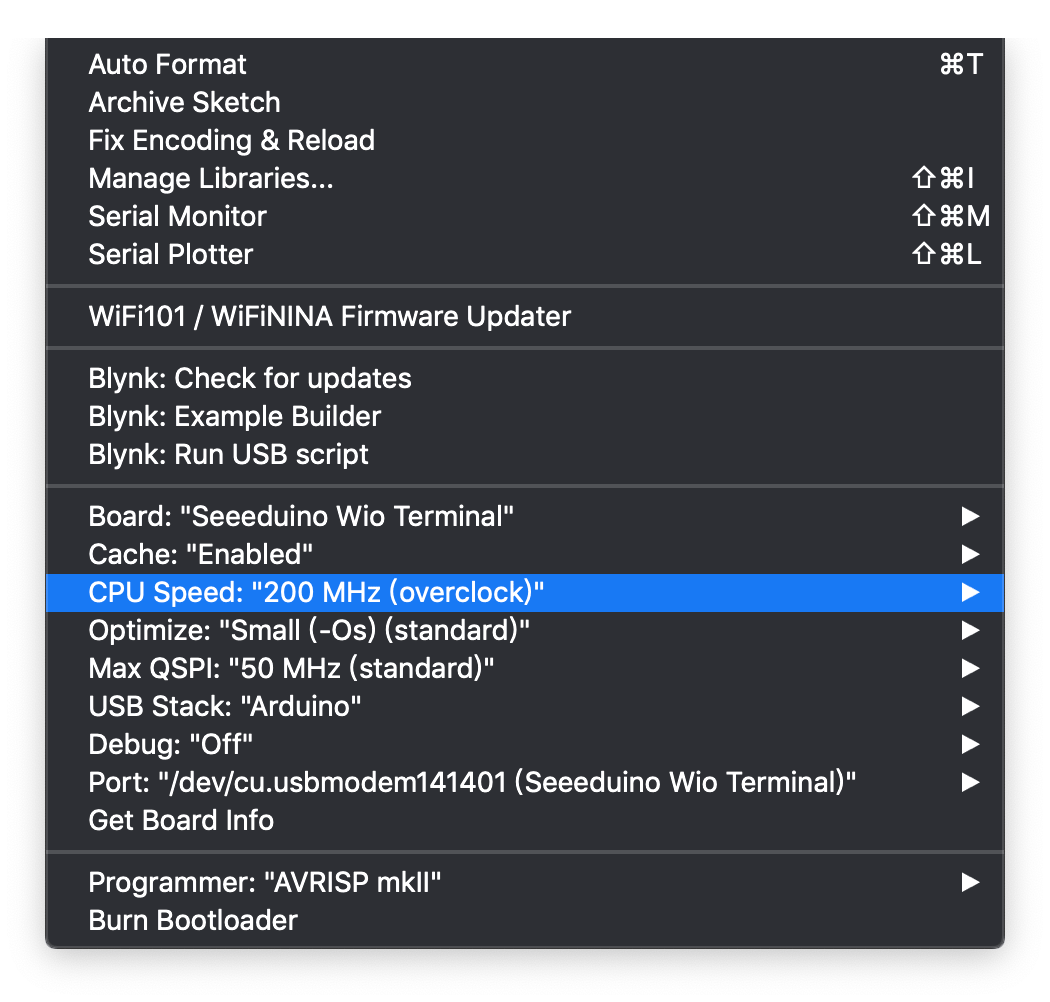# Build a IR Thermal Imaging Camera using Wio Terminal## Overview​

With Grove - Infrared Temperature Sensor Array (AMG8833) and Wio Terminal, we can build a low cost FLIR™ like Thermal Imagining camera with ease! To set you back a little, the resolution of the Grove - Infrared Temperature Sensor Array (AMG8833) is only 8 x 8 (64 Pixels), which in some cases is good enough. So Linear Interpolation is used in the code to expand to 70 x 70 (4900 Pixels) for a much better indications.

This demo is inspired by Kris Kasprzak's video. Several modifications were made to make it compatible with Wio Terminal and Grove - Infrared Temperature Sensor Array (AMG8833). Most Graphics are now first drawn to TFT LCD Sprites first to improve overall performance and faster frame rate. Also added a crosshair in the middle of screen and showing the temperature at the crosshair.

## Features​

• Indicating the exact temperature at crosshair

• IR Thermal Camera sensing thermal objects

• Right button to enable grid ON/OFF functionality

## Arduino libraries needed​

• Install the LCD screen Library `Seeed_Arduino_LCD`, please visit Wio Terminal LCD for more information.

• Now, the Seeed_AMG8833 library can be installed to the Arduino IDE. Open the Arduino IDE, and click `sketch` -> `Include Library` -> `Add .ZIP Library`, and choose the `Seeed_AMG8833` file that you've have just downloaded.

## Arduino Instructions​

• Plug in the the Grove - Infrared Temperature Sensor Array (AMG8833) to the Grove I2C Interface of Wio Terminal.

Note: To boost up performance and frame rate of this IR Thermal Imaging Camera, you can boost the Wio Terminal CPU Speed to 200MHz. Select `Tools` -> `CPU Speed` -> `200MHz(Overclock)```/*  This program is for upsizing an 8 x 8 array of thermal camera readings  it will size up by 10x and display to a 240 x 320  interpolation is linear and "good enough" given the display is a 5-6-5 color palet  Total final array is an array of 70 x 70 of internal points only  Revisions  1.0     Kasprzak      Initial code  1.1     Anson(Seeed Studio)  Adapted to Wio Terminal with Grove - Infrared Sensor(AMG8833)  */#include <Seeed_AMG8833_driver.h>#include <TFT_eSPI.h>                // Include the graphics library (this includes the sprite functions)  TFT_eSPI    tft = TFT_eSPI(); TFT_eSprite Display = TFT_eSprite(&tft);  // Create Sprite object "img" with pointer to "tft" object// the pointer is used by pushSprite() to push it onto the TFTunsigned long CurTime;uint16_t TheColor;// start with some initial colorsuint16_t MinTemp = 25;uint16_t MaxTemp = 35;// variables for interpolated colorsbyte red, green, blue;// variables for row/column interpolationbyte i, j, k, row, col, incr;float intPoint, val, a, b, c, d, ii;byte aLow, aHigh;// size of a display "pixel"byte BoxWidth = 3;byte BoxHeight = 3;int x, y;char buf;// variable to toggle the display gridint ShowGrid = -1;// array for the 8 x 8 measured pixelsfloat pixels;// array for the interpolated arrayfloat HDTemp;// create the camara objectAMG8833 ThermalSensor;//Toggle the grid on and offvoid toggleGrid() {  ShowGrid = ShowGrid *-1;  Display.fillRect(15, 15, 210, 210, TFT_BLACK);  yield();}void setup() {  Serial.begin(115200);  // start the display and set the background to black  tft.begin();  tft.fillScreen(TFT_BLACK);  //Interrupt to toggle Gird on and off  pinMode(WIO_KEY_A, INPUT);  attachInterrupt(digitalPinToInterrupt(WIO_KEY_A), toggleGrid, FALLING);  // set display rotation (you may need to change to 0 depending on your display  tft.setRotation(3);  // show a splash screen  tft.setCursor(20, 20);  tft.setTextColor(TFT_BLUE, TFT_BLACK);  tft.print("Thermal ");  tft.setTextColor(TFT_RED, TFT_BLACK);  tft.print("Camera");  // let sensor boot up  bool status = ThermalSensor.init();  delay(100);  if (!status) {      Serial.print("Failed to initalized AMG8833");  }  // read the camera for initial testing  ThermalSensor.read_pixel_temperature(pixels);  // check status and display results  if (pixels < 0) {    while (1) {      tft.setCursor(20, 40);      tft.setTextColor(TFT_RED, TFT_BLACK);      tft.print("Readings: FAIL");      delay(500);    }  }  else {    tft.setCursor(20, 40);    tft.setTextColor(TFT_GREEN, TFT_BLACK);    tft.print("Readings: OK");    delay(2000);  }  tft.fillScreen(TFT_BLACK);    Display.createSprite(TFT_HEIGHT, TFT_WIDTH);  Display.fillSprite(TFT_BLACK);   // get the cutoff points for the color interpolation routines  // note this function called when the temp scale is changed  Getabcd();  // draw a legend with the scale that matches the sensors max and min  DrawLegend();}void loop() {    CurTime = millis();  // draw a large white border for the temperature area  Display.fillRect(10, 10, 220, 220, TFT_WHITE);  // read the sensor  ThermalSensor.read_pixel_temperature(pixels);    // now that we have an 8 x 8 sensor array  // interpolate to get a bigger screen  // interpolate the 8 rows (interpolate the 70 column points between the 8 sensor pixels first)  for (row = 0; row < 8; row ++) {    for (col = 0; col < 70; col ++) {      // get the first array point, then the next      // also need to bump by 8 for the subsequent rows      aLow =  col / 10 + (row * 8);      aHigh = (col / 10) + 1 + (row * 8);      // get the amount to interpolate for each of the 10 columns      // here were doing simple linear interpolation mainly to keep performace high and      // display is 5-6-5 color palet so fancy interpolation will get lost in low color depth      intPoint =   (( pixels[aHigh] - pixels[aLow] ) / 10.0 );      // determine how much to bump each column (basically 0-9)      incr = col % 10;      // find the interpolated value      val = (intPoint * incr ) +  pixels[aLow];      // store in the 70 x 70 array      // since display is pointing away, reverse row to transpose row data      HDTemp[ (7 - row) * 10][col] = val;    }  }  // now that we have raw data with 70 columns  // interpolate each of the 70 columns  // forget Arduino..no where near fast enough..Teensy at > 72 mhz is the starting point  for (col = 0; col < 70; col ++) {    for (row = 0; row < 70; row ++) {      // get the first array point, then the next      // also need to bump by 8 for the subsequent cols      aLow =  (row / 10 ) * 10;      aHigh = aLow + 10;      // get the amount to interpolate for each of the 10 columns      // here were doing simple linear interpolation mainly to keep performace high and      // display is 5-6-5 color palet so fancy interpolation will get lost in low color depth      intPoint =   (( HDTemp[aHigh][col] - HDTemp[aLow][col] ) / 10.0 );      // determine how much to bump each column (basically 0-9)      incr = row % 10;      // find the interpolated value      val = (intPoint * incr ) +  HDTemp[aLow][col];      // store in the 70 x 70 array      HDTemp[ row ][col] = val;    }  }  //display the 70 x 70 array  DisplayGradient();  //Crosshair in the middle of the screen  Display.drawCircle(115, 115, 5, TFT_WHITE);  Display.drawFastVLine(115, 105, 20, TFT_WHITE);  Display.drawFastHLine(105, 115, 20, TFT_WHITE);  //Push the Sprite to the screen  Display.pushSprite(0, 0);  //Displaying the temp at the middle of the Screen  tft.setRotation(3);  tft.setTextColor(TFT_WHITE);  tft.drawFloat(HDTemp, 2, 90, 20);  //Uncomment this to print out frame rate  Serial.print("Frame rate: "); Serial.println(1/(0.001*(millis() - CurTime)));}// function to display the resultsvoid DisplayGradient() {  tft.setRotation(4);  // rip through 70 rows  for (row = 0; row < 70; row ++) {    // fast way to draw a non-flicker grid--just make every 10 pixels 2x2 as opposed to 3x3    // drawing lines after the grid will just flicker too much    if (ShowGrid < 0) {      BoxWidth = 3;    }    else {      if ((row % 10 == 9) ) {        BoxWidth = 2;      }      else {        BoxWidth = 3;      }    }    // then rip through each 70 cols    for (col = 0; col < 70; col++) {      // fast way to draw a non-flicker grid--just make every 10 pixels 2x2 as opposed to 3x3      if (ShowGrid < 0) {        BoxHeight = 3;      }      else {        if ( (col % 10 == 9)) {          BoxHeight = 2;        }        else {          BoxHeight = 3;        }      }      // finally we can draw each the 70 x 70 points, note the call to get interpolated color      Display.fillRect((row * 3) + 15, (col * 3) + 15, BoxWidth, BoxHeight, GetColor(HDTemp[row][col]));    }  }  }// my fast yet effective color interpolation routineuint16_t GetColor(float val) {  /*    pass in value and figure out R G B    several published ways to do this I basically graphed R G B and developed simple linear equations    again a 5-6-5 color display will not need accurate temp to R G B color calculation    equations based on    http://web-tech.ga-usa.com/2012/05/creating-a-custom-hot-to-cold-temperature-color-gradient-for-use-with-rrdtool/index.html  */  red = constrain(255.0 / (c - b) * val - ((b * 255.0) / (c - b)), 0, 255);  if ((val > MinTemp) & (val < a)) {    green = constrain(255.0 / (a - MinTemp) * val - (255.0 * MinTemp) / (a - MinTemp), 0, 255);  }  else if ((val >= a) & (val <= c)) {    green = 255;  }  else if (val > c) {    green = constrain(255.0 / (c - d) * val - (d * 255.0) / (c - d), 0, 255);  }  else if ((val > d) | (val < a)) {    green = 0;  }  if (val <= b) {    blue = constrain(255.0 / (a - b) * val - (255.0 * b) / (a - b), 0, 255);  }  else if ((val > b) & (val <= d)) {    blue = 0;  }  else if (val > d) {    blue = constrain(240.0 / (MaxTemp - d) * val - (d * 240.0) / (MaxTemp - d), 0, 240);  }  // use the displays color mapping function to get 5-6-5 color palet (R=5 bits, G=6 bits, B-5 bits)  return Display.color565(red, green, blue);}// function to get the cutoff points in the temp vs RGB graphvoid Getabcd() {  a = MinTemp + (MaxTemp - MinTemp) * 0.2121;  b = MinTemp + (MaxTemp - MinTemp) * 0.3182;  c = MinTemp + (MaxTemp - MinTemp) * 0.4242;  d = MinTemp + (MaxTemp - MinTemp) * 0.8182;}// function to draw a legendvoid DrawLegend() {  //color legend with max and min text  j = 0;  float inc = (MaxTemp - MinTemp ) / 160.0;  for (ii = MinTemp; ii < MaxTemp; ii += inc) {    tft.drawFastHLine(260, 200 - j++, 30, GetColor(ii));  }  tft.setTextSize(2);  tft.setCursor(245, 20);  tft.setTextColor(TFT_WHITE, TFT_BLACK);  sprintf(buf, "%2d/%2d", MaxTemp, (int) (MaxTemp * 1.8) + 32);  tft.print(buf);  tft.setTextSize(2);  tft.setCursor(245, 210);  tft.setTextColor(TFT_WHITE, TFT_BLACK);  sprintf(buf, "%2d/%2d", MinTemp, (int) (MinTemp * 1.8) + 32);  tft.print(buf);}// END OF CODE``